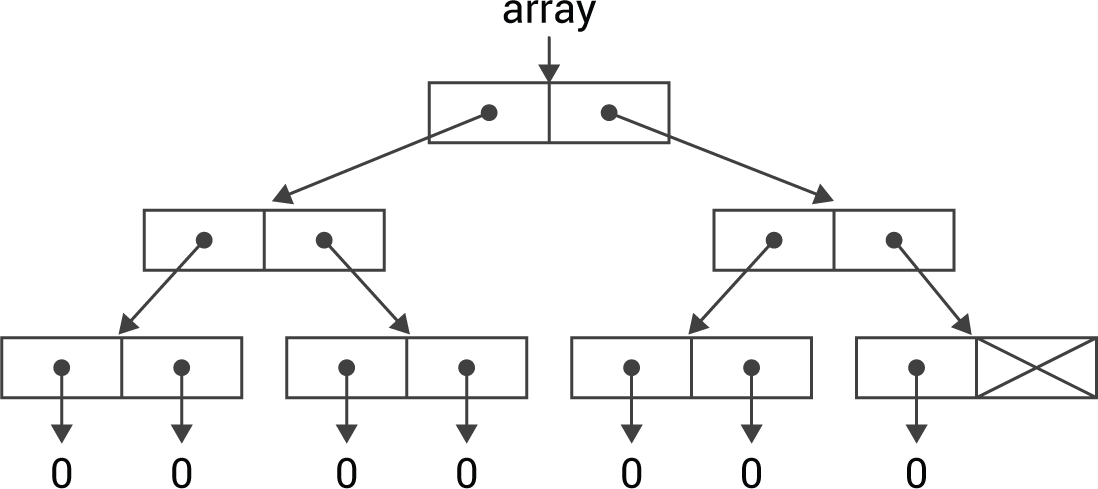## Arrays

Version 3.2 of uLisp added multidimensional arrays to the 32-bit versions of uLisp, and Version 3.3 extended the array support to include bit arrays.

uLisp's implementation supports arrays with an arbitrary number of dimensions, and packs bit arrays as efficiently as possible, so they take up a factor of 32 less storage than the equivalent-sized integer array.

### Implementation

The traditional way to implement an array is as a block of contiguous memory, so the address of an array element can simply be calculated as an offset from the base address of the array.

However, this approach would have been difficult with uLisp. It would require a separate block of memory to be allocated for array storage, and a separate garbage collection mechanism would be needed for arrays.

Instead I decided to use the existing uLisp memory model by implementing arrays using a binary tree. This could use the existing memory allocation system, and would be able to use the same garbage collector.

This wouldn’t be as efficient as a conventional array, using sequential memory, but it would still be a big improvement over using a list with nth. For example, a 1024-element array would require 10 steps to index to an element rather than, on average, 512 when using a list.

### Building an array

The function that creates a new array structure in the workspace is buildarray(); this takes three parameters:

• n - the size of the array
• s - the size of the binary tree, which is a power of two
• def - the default value for each array element

The size s is the smallest power of 2 that is equal to or greater than n. It's calculated by the routine nextpower2():

```int nextpower2 (int n) {
n--; n |= n >> 1; n |= n >> 2; n |= n >> 4;
n |= n >> 8; n |= n >> 16; n++;
return n<2 ? 2 : n;
}```

Here's the definition of buildarray():

```object *buildarray (int n, int s, object *def) {
int s2 = s>>1;
if (s2 == 1) {
if (n == 2) return cons(def, def);
else if (n == 1) return cons(def, NULL);
else return NULL;
} else if (n >= s2) return cons(buildarray(s2, s2, def), buildarray(n - s2, s2, def));
else return cons(buildarray(n, s2, def), nil);
}```

For example, the call:

`object *array = buildarray(7, 8, zero);`

where zero is a pointer to the Lisp number zero, will create:### Getting an array reference

The function arrayref() returns a pointer to a particular element in an array:

```object **arrayref (object *array, int index, int size) {
object **p = &car(cdr(array));
if ((index & mask) == 0) p = &(car(*p)); else p = &(cdr(*p));
}
return p;
}```

The parameters are as follows:

• array is the array object
• index is the array element to be returned
• size is the number of elements in the array

It returns a pointer to the array element, rather than the element itself, so the same routine can be used to modify an array element with a Lisp statement such as:

`(setf (aref a 6) "Hello")`

The routine arrayref() works as follows: for each of the bits in index starting with the nth, where n is the number of bits needed to represent size, follow down the tree, taking the left branch if the bit is a '0', or the right path if the bit is a '1'. The final cell will be the array element specified by index.

### Other routines

The following additional routines are provided for working with arrays:

makearray() builds an array with an arbitrary list of dimensions by calculating the total size, and then calling buildarray(). It also handles bit arrays.

getarray() returns a pointer to an element in a multi-dimensional array, given a list of the subscripts, by calling arrayref().

rslice() recursively reads a slice of an array.